## General Question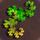# What does this short snippet of C code do? (see details)

Asked by PhiNotPi (12680) February 19th, 2012

I have a short piece of C code that I am having trouble understanding (I don’t know much about C).

My best guess for what it does is that it opens a random number generator to make a random number “val” inside of a range given by “n”.

`handle = open(”/dev/urandom”, O_RDONLY);`
`do {`
`read(handle, &bits, sizeof bits);`
`bits &= 0×7fffffff;`
`val = bits % n;`
`} while (bits – val + (n-1) < 0);`
`close(handle);`

What I am looking for is a line-by-line, maybe even a word-by-word analysis of what it does and why, because I don’t have a clue what some of it does. I pretty sure that the answer is very straightforward, it is just that I don’t know what it is.

Observing members: 0Composing members: 0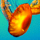It’s been a long time since I did much in C… but

It looks like it reads a file (or url?) and assigns that reference to the variable “handle” to use later, then reads each bit or line of the file and once it’s been through the whole file closes the reference.

By itself, it doesn’t do anything really except read the file and there are some variables that are defined outside the snippet you have here… (n, sizeof bits), some of these may be constants used in C.

I’d guess this is a function just meant to return file contents in an expected format for use elsewhere. More of the context would provide more clues.

funkdaddy (17772)“Great Answer” (1) Flag as…@funkdaddy The context is that it is part of a program that outputs a single number based on another number and a RNG. Here is where the variables are declared:
`int32_t bits, val, n = 0;`

PhiNotPi (12680)“Great Answer” (0) Flag as…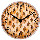Likewise, it’s been while since I did anything in C. But I can give you a partial answer.

handle = open(”/dev/urandom”, O_RDONLY); Open the file urandom in folder dev for read only

do { Enclose the following statements in a loop

read(handle, &bits, sizeof bits); Read the file urandom (via the variable handle) into a memory location pointed to by the variable bits

bits &= 0×7fffffff; This one, I’m not sure what it’s doing. It looks like it’s storing hex value 7FFFFFFF in the memory location named bits. But I don’t remember what the &= means. Something weird with pointers probably, but I’m not sure.

val = bits % n; compute val as the modulus (bits / n)

} while (bits – val + (n-1) < 0); Repeat the loop while bits-val+(n-1) < 0. But your code doesn’t say what n is initialized to, so that’s an unknown.

close(handle); close the file, take your toys, and go home

HungryGuy (16029)“Great Answer” (1) Flag as…@HungryGuy I looked up ”&=” and it seems to be a bitwise AND operation. This makes me wonder even more, because 7fffffff is a string of all ones.

PhiNotPi (12680)“Great Answer” (0) Flag as…Okay. Here’s an example of a boolean AND operation:

1010
1100 and
——
1000

So ANDing something with 1 yields the original value. So AND 7FFFFFFF is forcing the first bit to be 0 while passing the rest unchanged.

HungryGuy (16029)“Great Answer” (2) Flag as…@HungryGuy 7FFFFFFF seems to be a string of 32 ones, so it seems like the AND operation wouldn’t do anything.

One theory that I have is that it limits the length of the bit stream by only allowing 32 bits through.

PhiNotPi (12680)“Great Answer” (1) Flag as…Look at it in binary:

7FFFFFFF = 0111 1111 1111 1111 1111 1111 1111 11111

So ANDing someting with this value will force the first bit to be zero while passing the other bits unchanged.

HungryGuy (16029)“Great Answer” (2) Flag as…Just guessing I’d say the file has a string of random numbers, it reads in a group of them in as a kind of salt, then adds the max to it (0×7FFFFFFF is hexadecimal for the max integer I believe).

I’d guess &= is similar to += or .= in other languages, basically “take what’s already there, and add this to it”

So we have a big number plus our string of numbers (read as an integer) divided by N (probably our input number?) and we just want the remainder, which will always be positive.

Then we check our big number (bits) minus our new remainder, plus our entered number minus one for some reason, and make sure that’s positive before we move on.

So I’d say they return val and want to make sure it’s not larger than bits essentially.

Stackoverflow or something similar could probably get you a real deal C programmer who isn’t guessing. Might be a good next stop if you need more.

funkdaddy (17772)“Great Answer” (1) Flag as…@HungryGuy Oh, it’s only 31 ones.

PhiNotPi (12680)“Great Answer” (1) Flag as…Right. And at this point, I have to agree with @funkdaddy: you need someone who is up to speed in C.

HungryGuy (16029)“Great Answer” (1) Flag as…@HungryGuy I am probably going to go ask this at StackOverflow. I guess Fluther is just not the place for programming help.

PhiNotPi (12680)“Great Answer” (1) Flag as…@PhiNotPi – At least you didn’t ask for medical advice for some obscure condition :-p

HungryGuy (16029)“Great Answer” (2) Flag as…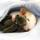Here is an explanation of /dev/urandom.

ratboy (15167)“Great Answer” (2) Flag as…It reads into bits and keeps the last 31 bits.
val = bits mod n. I am guessing that n is a power of 2, 2^k for some k. That would have val containing the last k bits of bits.

The while determines a condition that will cause another read unless the condition is violated.
(bits – val) equals bits with the last k bits set to 0. n – 1 is one less than 2^k (using my guess), so it is equal to k one’s. Thus adding n – 1 equals bits with the last k bits set to 1.

Here it gets confusing. Assuming that bits is a single precision 32 bit value, being less than 0 means that the 32nd bit equals 1 (-x is stored in computers as 2^32 – x). Since the number does not contain the 32nd bit, I don’t see how this condition will ever be true.

LostInParadise (29659)“Great Answer” (2) Flag as…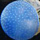It looks like the guts under the system function rand().

@ratboy‘s including the definition of /dev/random is very useful. Clearly that’s a system-wide base string for pseudo-random operations.
This function starts by reading a ‘sizeof’ chunk of whatever’s in that file. In this case sizeof is 32 bits per the definition of “bits” (int32_t bits).

“bits &= 0×7fffffff;” just strips the top bit from the fetched string, limiting the ‘bits’ value to positive integers. (per @funkdaddy‘s analysis) This seems superfluous since we only got 32 bits in the first place….

I don’t believe “n = 0”, maybe it is at initialization, but if it is zero the modulus is always zero (val = bits % n;) which makes the whole thing pretty useless. I think you will pass n into the function as a ‘range’ or max size of the random number you want from the function.
[ e.g. if you have 17 students and want to select one at random you pass 17 as ‘n’ into the function and the result will be somewhere between 1 and 17 ]

dabbler (18865)“Great Answer” (0) Flag as…If bits = q*n + r where 0 <= r < |n|, then val = r and bits – val + (n – 1) is q*n + r – r + n – 1 = (q + 1)*n – 1. The condition being tested is (q + 1)*n < 1. If, as seems reasonable, bits is a random bit string coerced into an integer with the sign bit cleared, then this condition is satisfied only when n < 0 and |n| <= bits < |2*n|.

ratboy (15167)“Great Answer” (0) Flag as…or